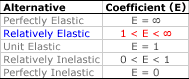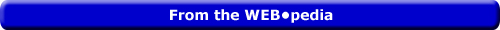Tuesday  August 9, 2022
 AmosWEB means Economics with a Touch of Whimsy!TOTAL COST AND MARGINAL COST: A mathematical connection between marginal cost and total cost stating that marginal cost IS the slope of the total cost curve. If the total cost curve has a positive slope (that is, is upward sloping), then marginal cost is positive. Moreover, if the total cost curve has a positive and increasingly steeper slope, then the marginal cost is positive and rising. If the total cost curve has a positive and decreasingly steeper slope, then the marginal cost is positive but falling.Lesson 11: Elasticity Basics | Unit 4: A Continuum Page: 18 of 25

 Topic: Relative Elastic <=PAGE BACK | PAGE NEXT=>• A large section of the elasticity continuum is comprise of relatively elastic:

Relatively elastic is an elasticity alternative in which relatively small changes in one variable (price) cause relatively large changes in another variable (quantity).

• Once again, this range holds for both the price elasticity of demand and the price elasticity of supply, because the negative value of the price elasticity of demand is ignored.

• Relatively elastic demand and supply are represented by relatively flat, but not perfectly horizontal, demand and supply curves.

| | |PERFECTLY ELASTIC

An elasticity alternative in which infinitesimally small changes in one variable (usually price) cause infinitely large changes in another variable (usually quantity). Quantity is infinitely responsive to price. Any change in price, no matter how small, triggers an infinite change in quantity. This characterization of elasticity is most important for the price elasticity of demand and the price elasticity of supply. Perfectly elastic is one of five elasticity alternatives. The other four are perfectly inelastic, relatively elastic, relatively inelastic, and unit elastic.GREEN LOGIGUIN[What's This?] Today, you are likely to spend a great deal of time strolling around a discount warehouse buying club trying to buy either a birthday gift for your uncle or a pair of red and purple designer socks. Be on the lookout for the last item on a shelf.Your Complete ScopeNatural gas has no odor. The smell is added artificially so that leaks can be detected."Work is an extension of personality. It is achievement. It is one of the ways in which a person defines himself, measures his worth ‚ and his humanity. "-- Peter Drucker, authorAPAverage ProductA PEDestrian's Guide Xtra CreditTell us what you think about AmosWEB. Like what you see? Have suggestions for improvements? Let us know. Click the User Feedback link.| | | | | | | | | | |
| | | |

Thanks for visiting AmosWEB